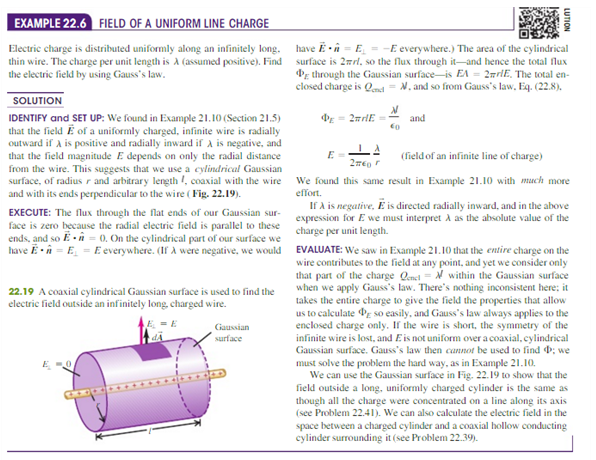# Problem: An infinitely long cylindrical conductor has radius R and uniform surface charge density σ. (a) In terms of σ and , what is the charge per unit length λ for the cylinder? (b) In terms of s, what is the magnitude of the electric field produced by the charged cylinder at a distance r  &gt; R from its axis? (c) Express the result of part (b) in terms of λ and show that the electric field outside the cylinder is the same as if all the charge were on the axis. Compare your result to the result for a line of charge in Example 22.6 (Section 22.4).

###### FREE Expert Solution

Electric flux through a closed surface, from Gauss's law, is expressed as:

$\overline{){{\mathbf{\varphi }}}_{{\mathbf{E}}}{\mathbf{=}}{\mathbf{\int }}\stackrel{\mathbf{⇀}}{\mathbf{E}}{\mathbf{·}}{\mathbf{d}}\stackrel{\mathbf{⇀}}{\mathbf{s}}{\mathbf{=}}\frac{\mathbf{Q}}{{\mathbf{\epsilon }}_{\mathbf{0}}}}$

(a)

Considering a cylinder of infinite length and imagining that a cylinder of finite length, l, is cut from this cylinder.

92% (205 ratings)###### Problem Details

An infinitely long cylindrical conductor has radius R and uniform surface charge density σ.

(a) In terms of σ and , what is the charge per unit length λ for the cylinder?

(b) In terms of s, what is the magnitude of the electric field produced by the charged cylinder at a distance r  > R from its axis?

(c) Express the result of part (b) in terms of λ and show that the electric field outside the cylinder is the same as if all the charge were on the axis. Compare your result to the result for a line of charge in Example 22.6 (Section 22.4).